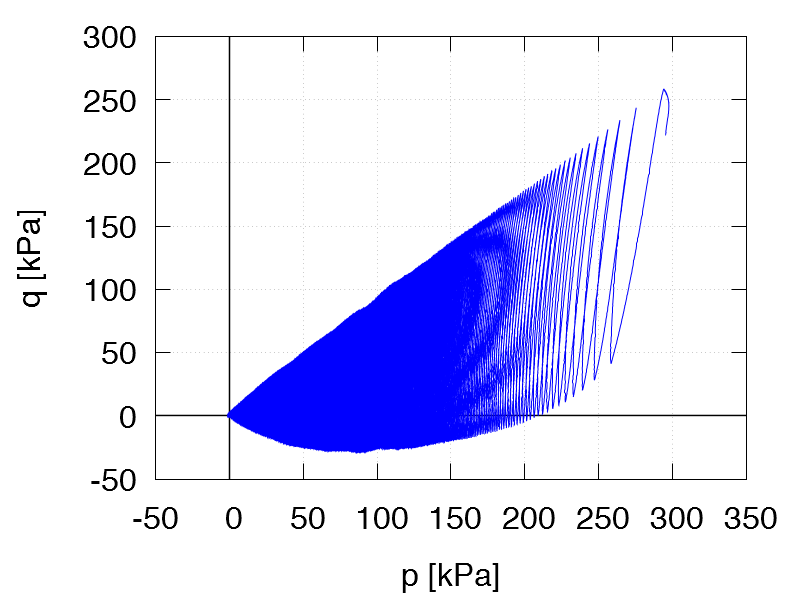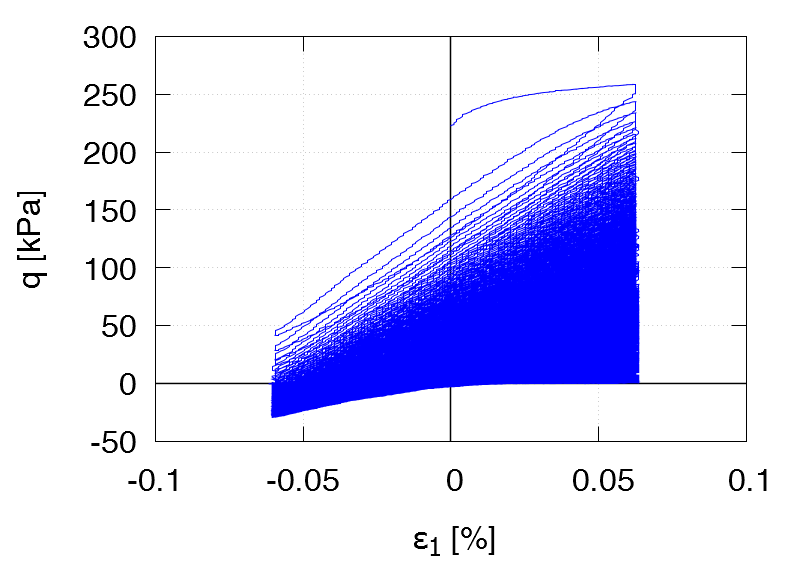Undrained cyclic tests with strain cycles of small amplitude:   Data of all tests of this series
 TCUE1       ID0 = 0.64 p0 = 200 kPa η0 = 0.75 ε1ampl = 6.0 ⋅ 10-4 Test data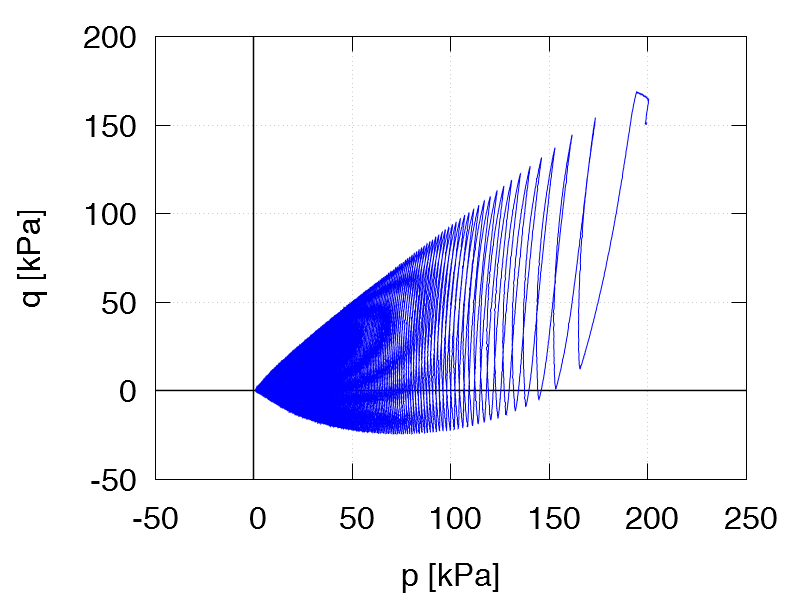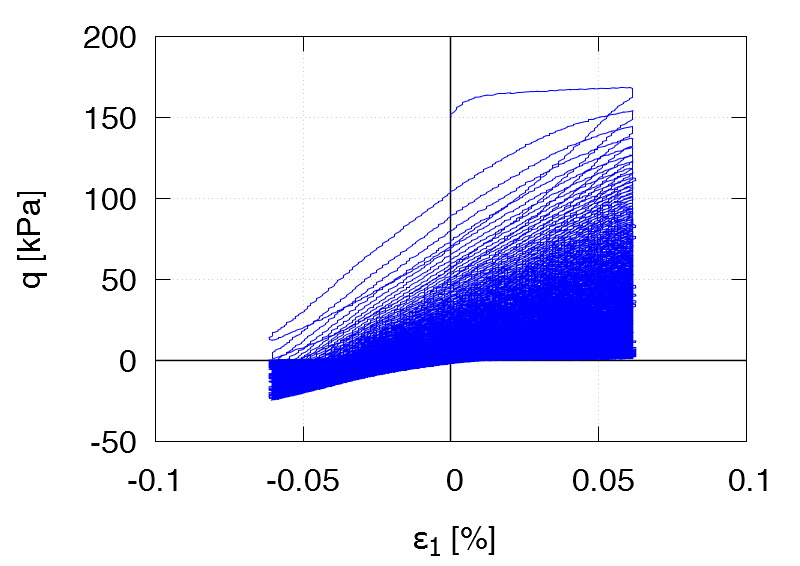TCUE2 ID0 = 0.70 p0 = 200 kPa η0 = 0.75 ε1ampl = 6.0 ⋅ 10-4 1st drained cycle Test data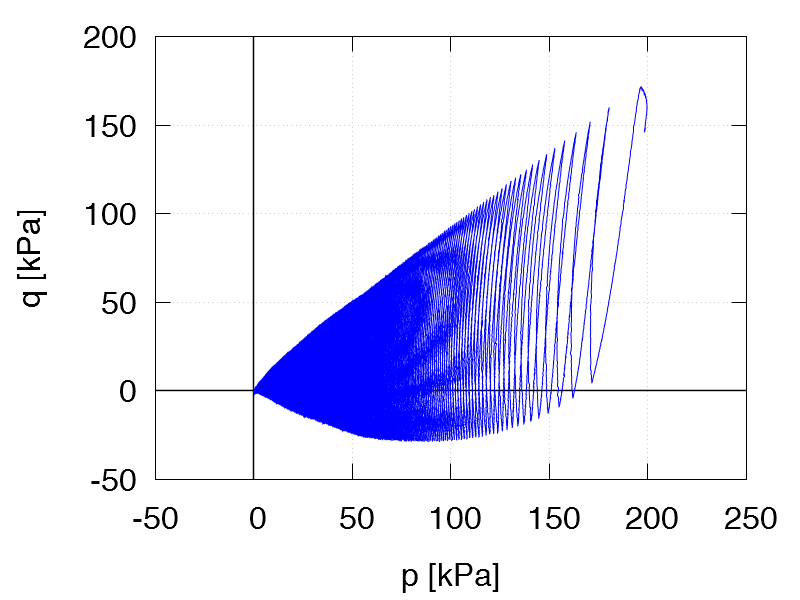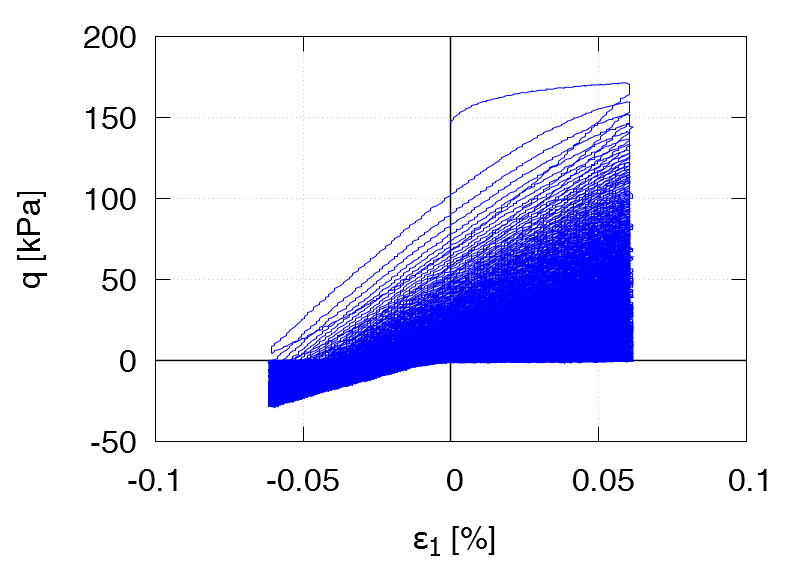TCUE3 ID0 = 0.53 p0 = 200 kPa η0 = -0.5 ε1ampl = 6.0 ⋅ 10-4 Test data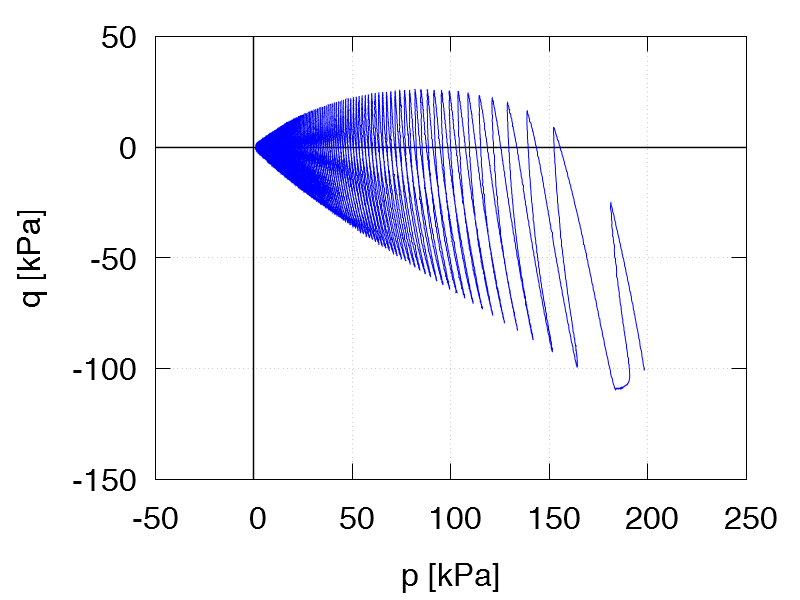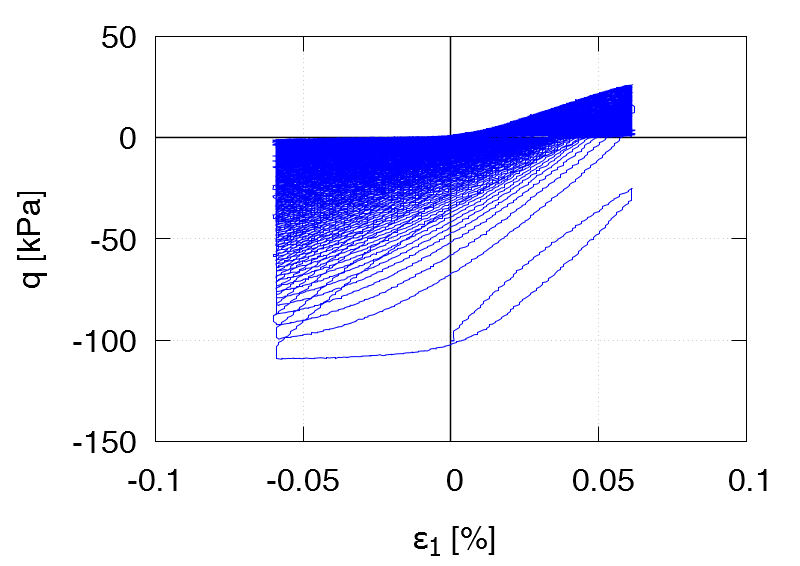TCUE4 ID0 = 0.60 p0 = 200 kPa η0 = 0.75 ε1ampl = 4.0 ⋅ 10-4 1st drained cycle Test data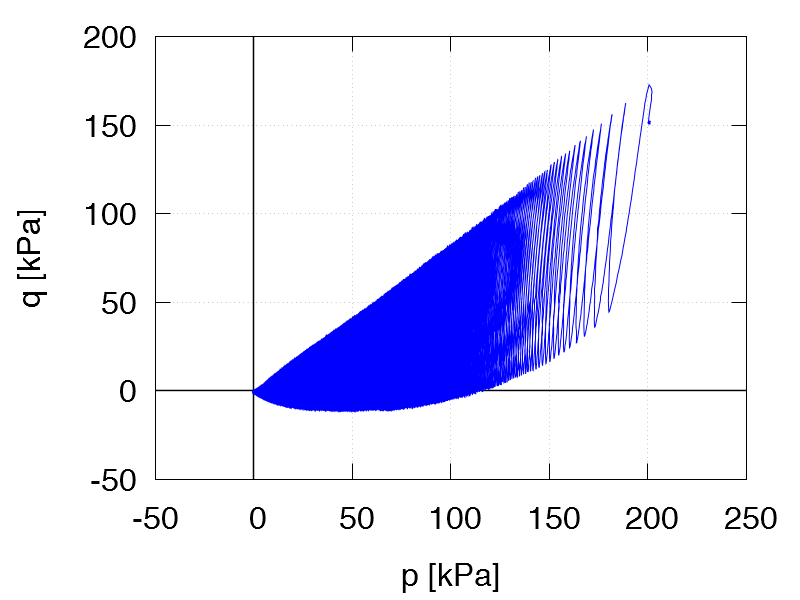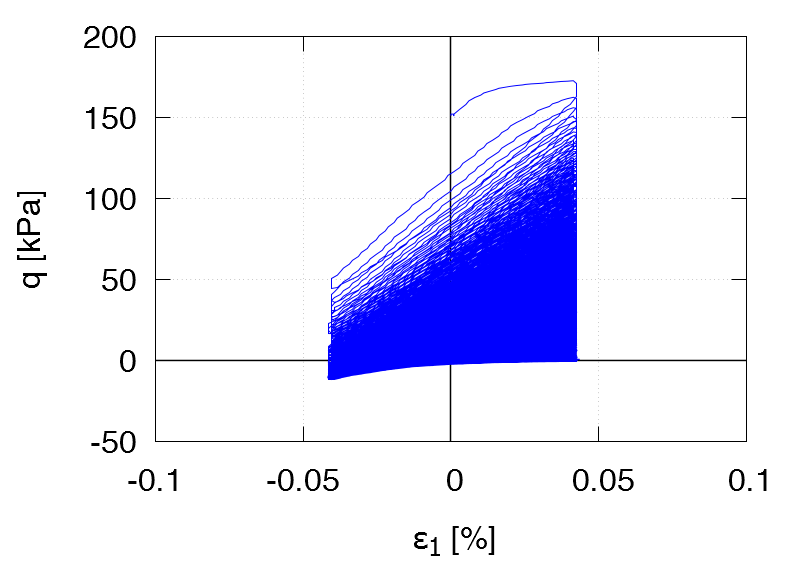TCUE5 ID0 = 0.69 p0 = 200 kPa η0 = 0.75 ε1ampl = 8.0 ⋅ 10-4 1st drained cycle Test data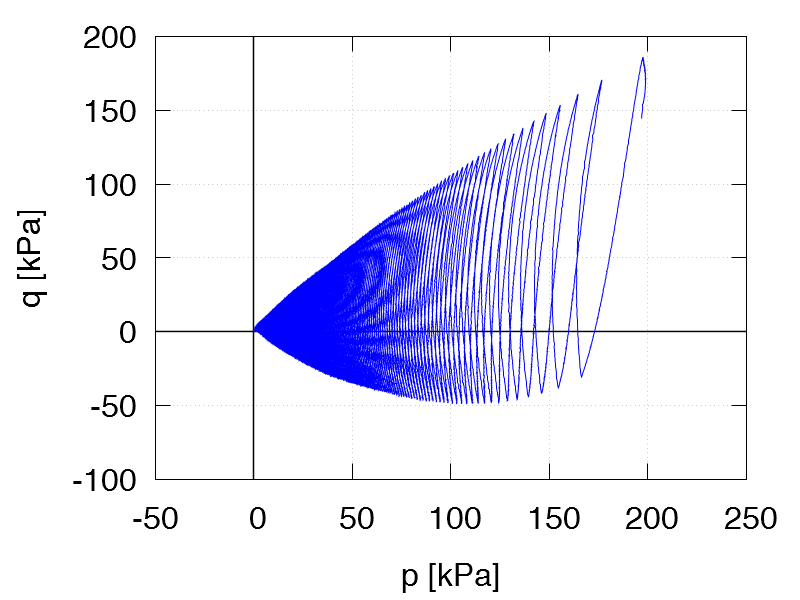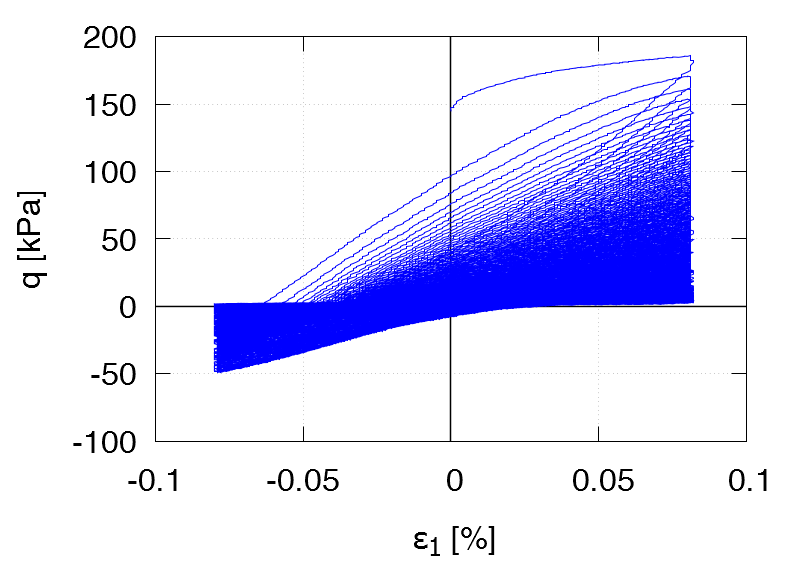TCUE6 ID0 = 0.38 p0 = 200 kPa η0 = 0.75 ε1ampl = 6.0 ⋅ 10-4 1st drained cycle Test data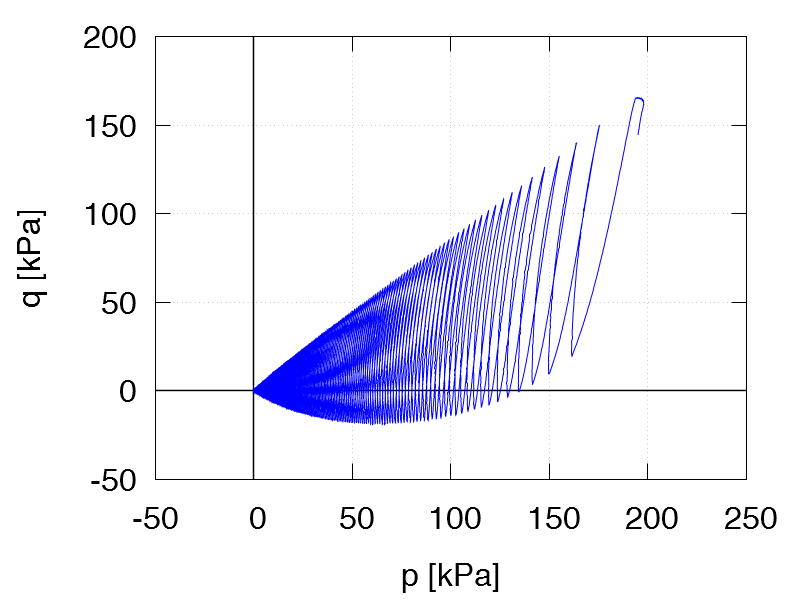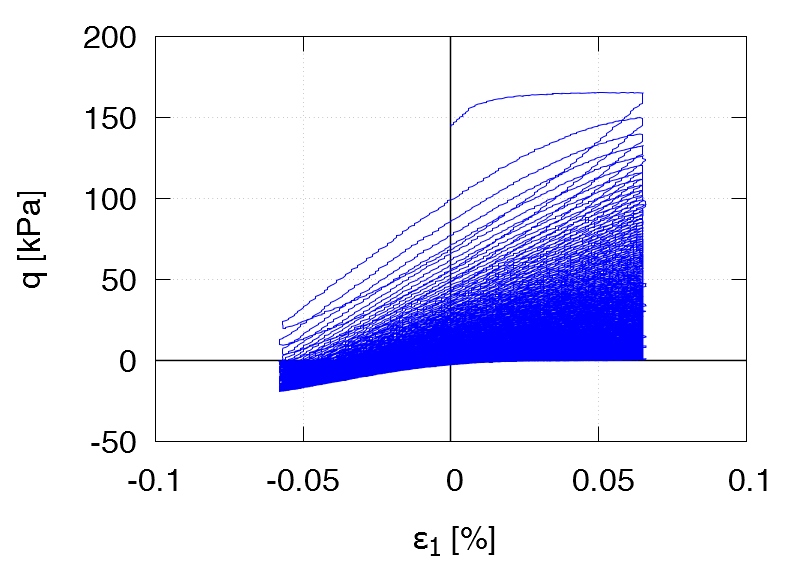TCUE7 ID0 = 0.82 p0 = 200 kPa η0 = 0.75 ε1ampl = 6.0 ⋅ 10-4 1st drained cycle Test data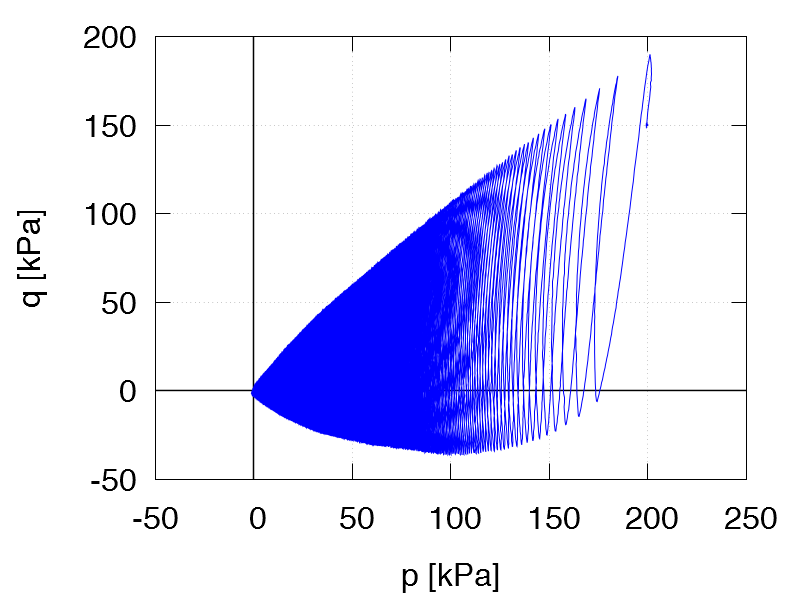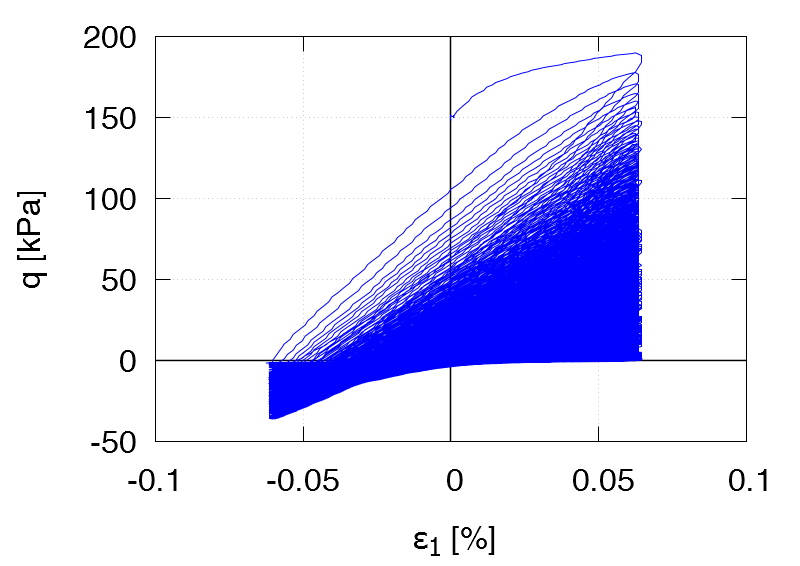TCUE8 ID0 = 0.66 p0 = 200 kPa η0 = 1.15 ε1ampl = 6.0 ⋅ 10-4 1st drained cycle Test data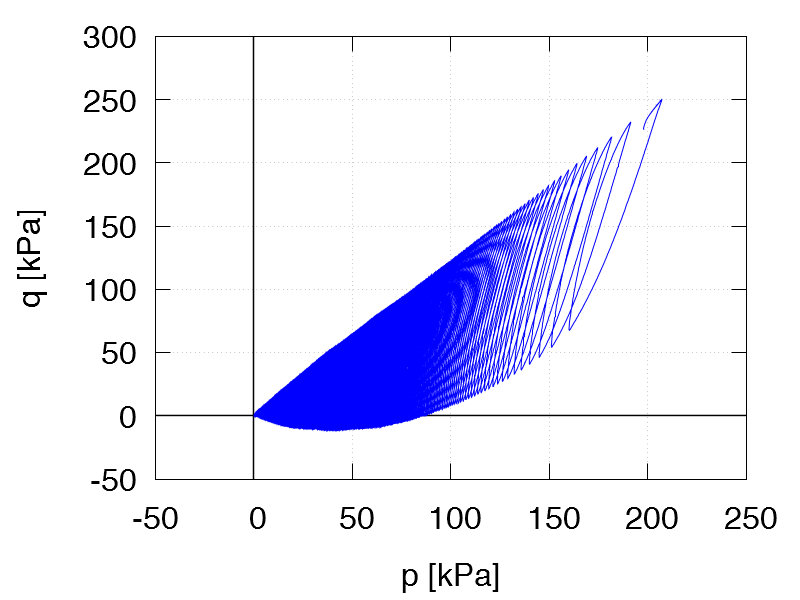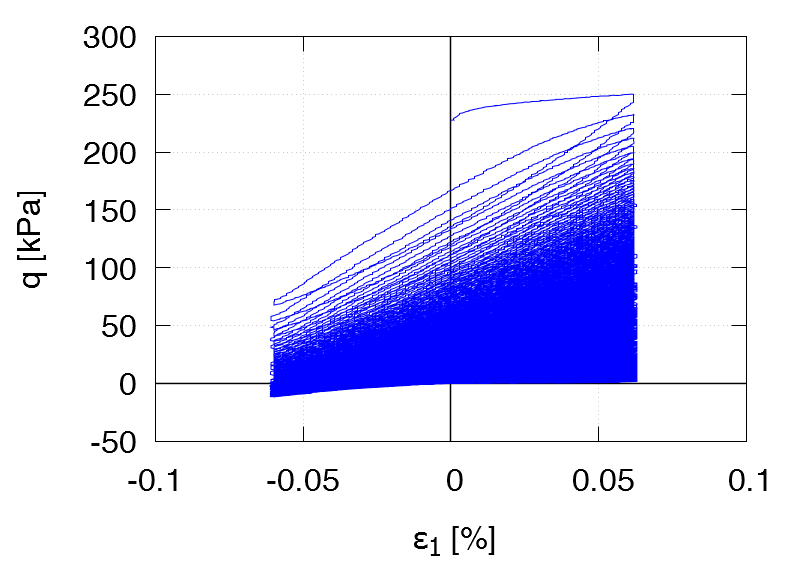TCUE9 ID0 = 0.67 p0 = 200 kPa η0 = 0 ε1ampl = 6.0 ⋅ 10-4 1st drained cycle Test data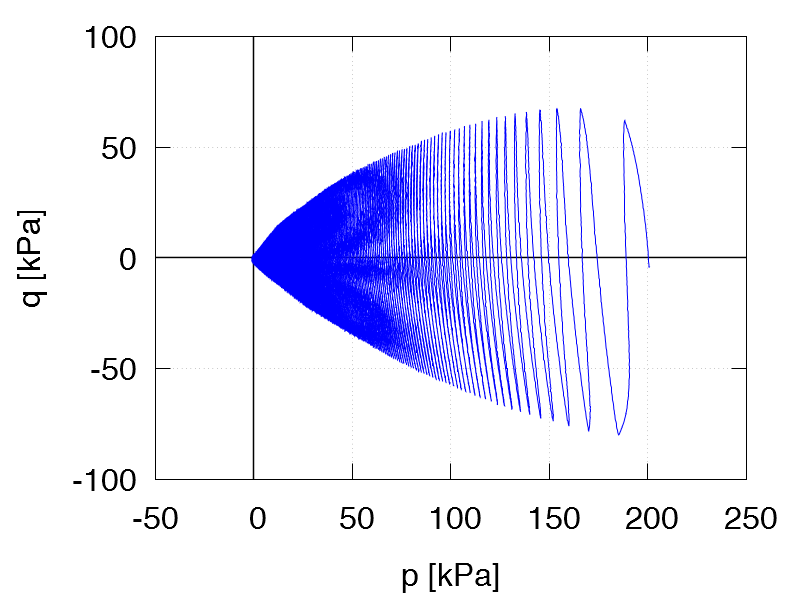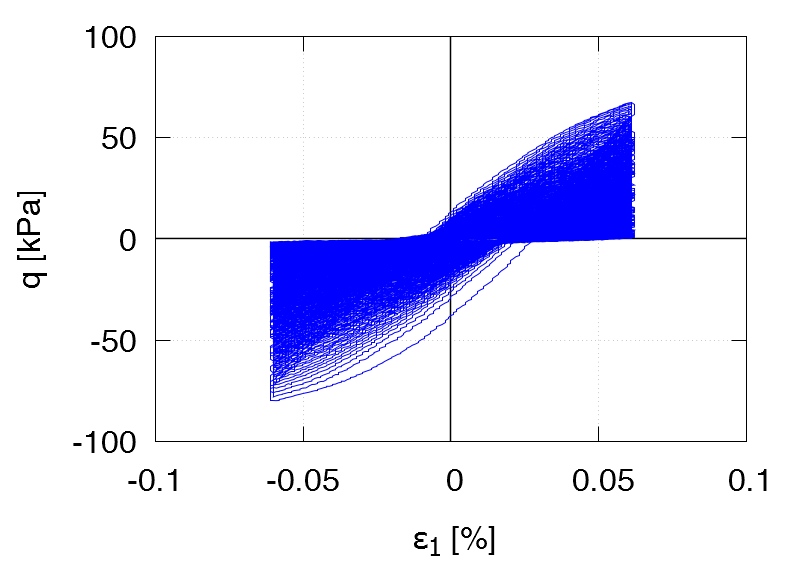TCUE10 ID0 = 0.56 p0 = 200 kPa η0 = -0.5 ε1ampl = 6.0 ⋅ 10-4 1st drained cycle Test data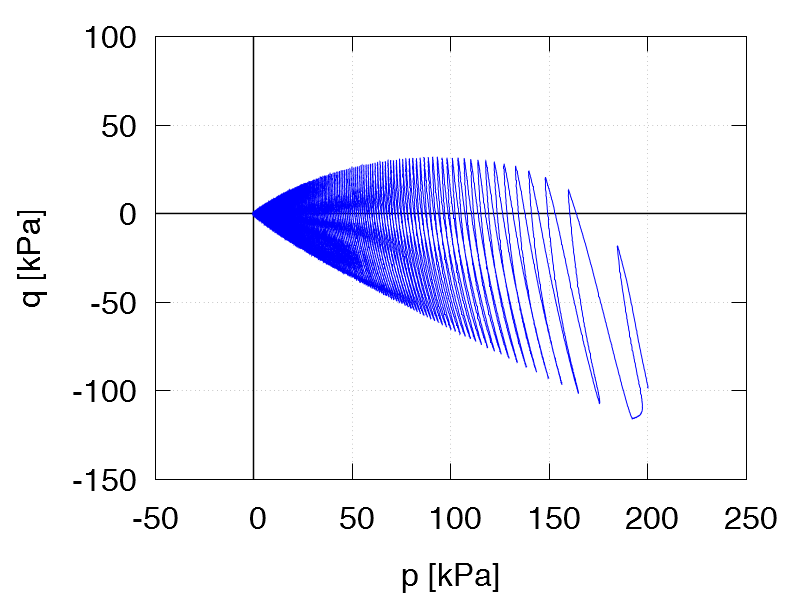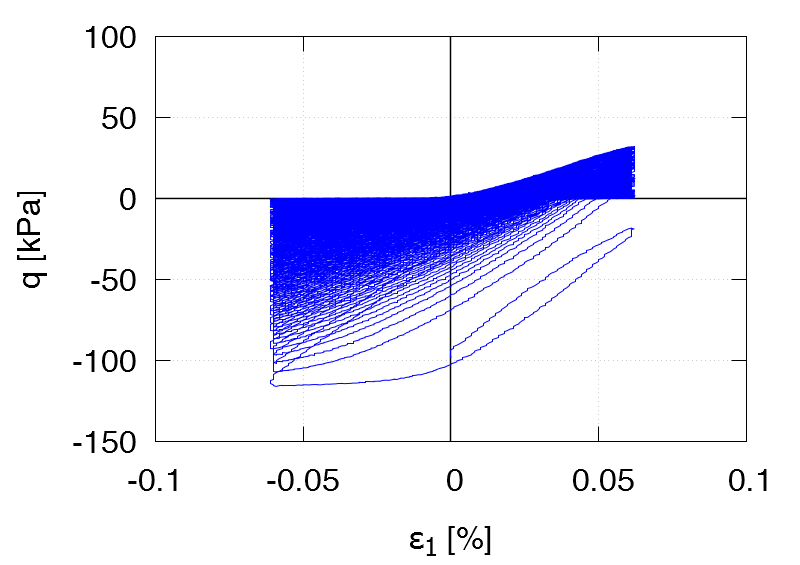TCUE11 ID0 = 0.58 p0 = 200 kPa η0 = -0.75 ε1ampl = 6.0 ⋅ 10-4 1st drained cycle Test data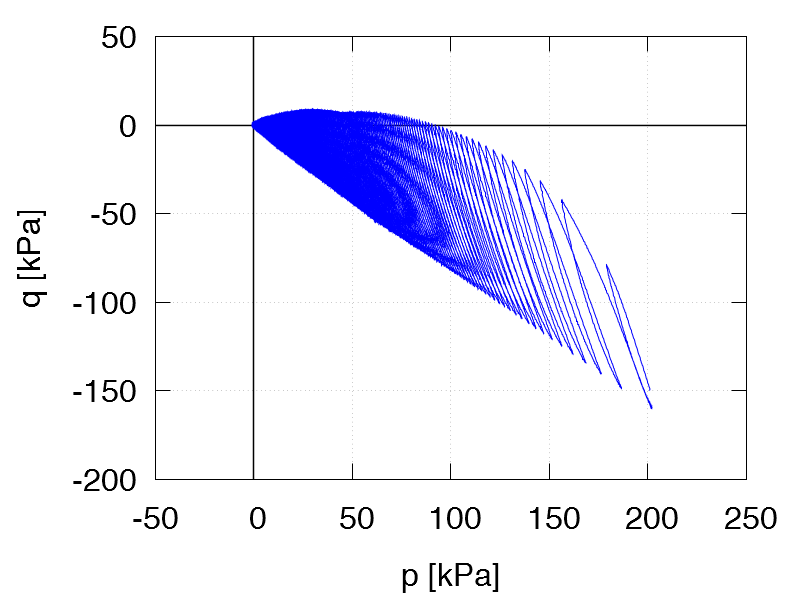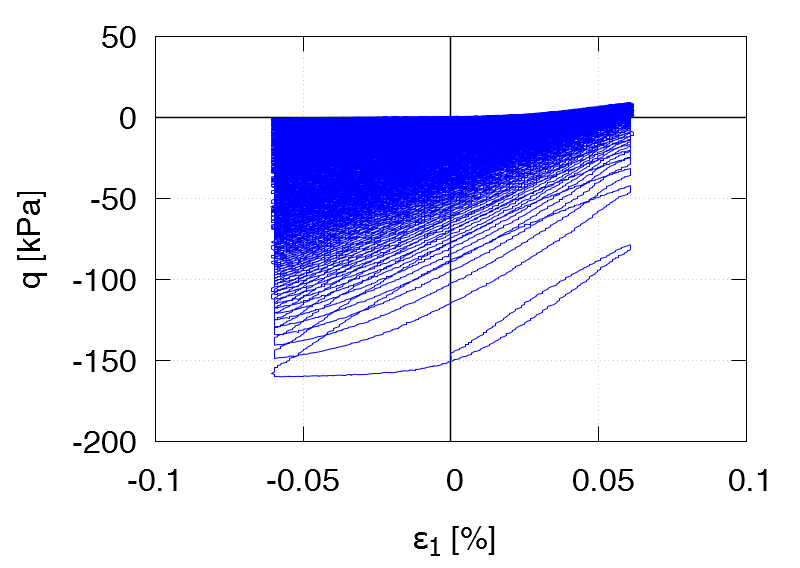TCUE12 ID0 = 0.59 p0 = 50 kPa η0 = 0.75 ε1ampl = 6.0 ⋅ 10-4 1st drained cycle Test data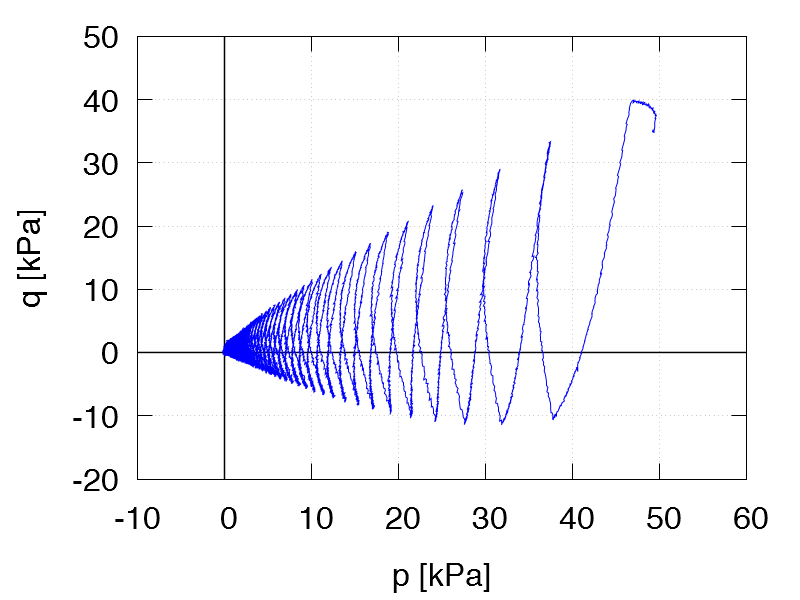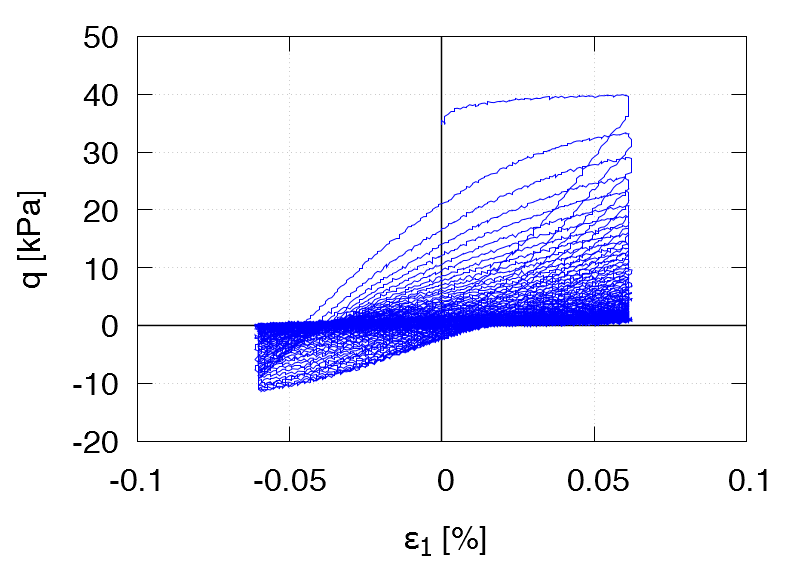TCUE13 ID0 = 0.71 p0 = 100 kPa η0 = 0.75 ε1ampl = 6.0 ⋅ 10-4 1st drained cycle Test data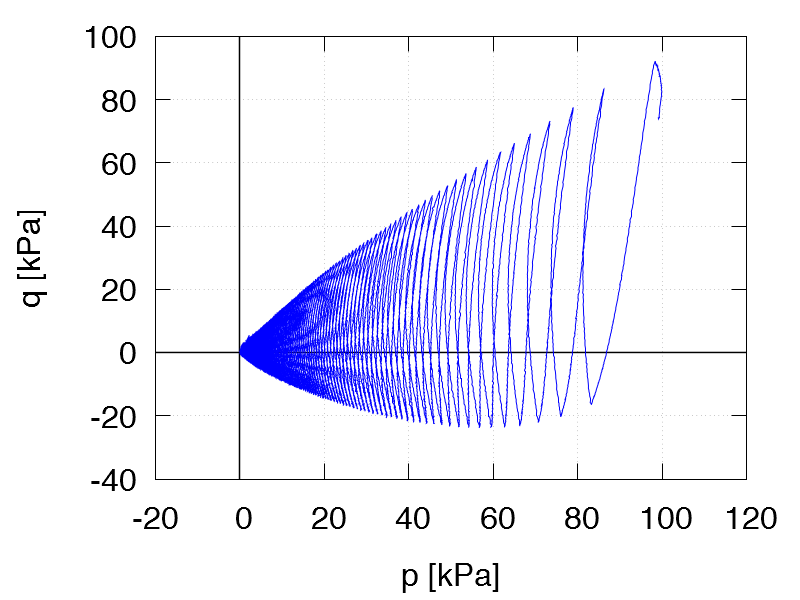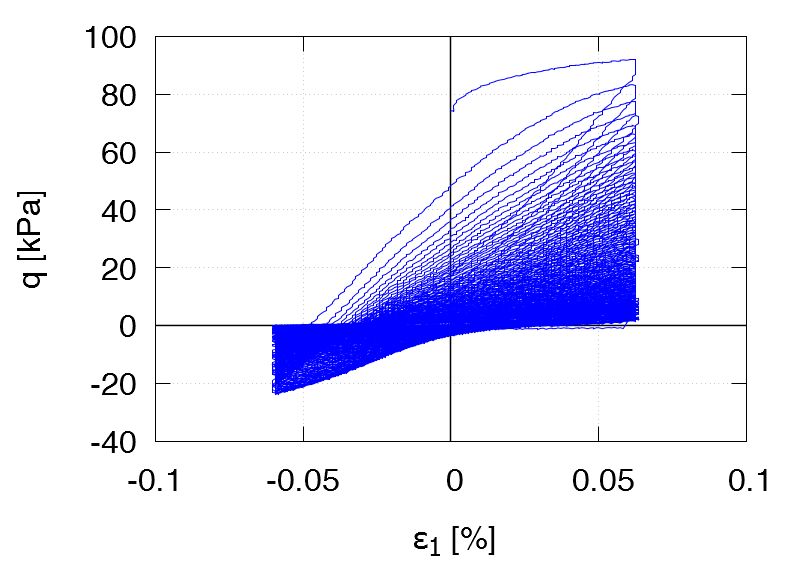TCUE14 ID0 = 0.66 p0 = 300 kPa η0 = 0.75 ε1ampl = 6.0 ⋅ 10-4 1st drained cycle Test data